# IPython Interactive Computing and Visualization Cookbook - Second Edition4.5 (4 reviews total)
By Cyrille Rossant
• \$28.99 eBook
• \$36.99 Print + eBook
What do you get with a Packt Subscription?

• Instant access to this title and 7,500+ eBooks & Videos
• Constantly updated with 100+ new titles each month
• Breadth and depth in over 1,000+ technologies

Python is one of the leading open source platforms for data science and numerical computing. IPython and the associated Jupyter Notebook offer efficient interfaces to Python for data analysis and interactive visualization, and they constitute an ideal gateway to the platform.

IPython Interactive Computing and Visualization Cookbook, Second Edition contains many ready-to-use, focused recipes for high-performance scientific computing and data analysis, from the latest IPython/Jupyter features to the most advanced tricks, to help you write better and faster code. You will apply these state-of-the-art methods to various real-world examples, illustrating topics in applied mathematics, scientific modeling, and machine learning.

The first part of the book covers programming techniques: code quality and reproducibility, code optimization, high-performance computing through just-in-time compilation, parallel computing, and graphics card programming. The second part tackles data science, statistics, machine learning, signal and image processing, dynamical systems, and pure and applied mathematics.

Publication date:
January 2018
Publisher
Packt
Pages
548
ISBN
9781785888632

## Chapter 1. A Tour of Interactive Computing with Jupyter and IPython

In this chapter, we will cover the following topics:

• Introducing IPython and the Jupyter Notebook

• Getting started with exploratory data analysis in the Jupyter Notebook

• Introducing the multidimensional array in NumPy for fast array computations

• Creating an IPython extension with custom magic commands

• Mastering IPython's configuration system

• Creating a simple kernel for Jupyter

## Introduction

In this introduction, we will give a broad overview of Python, IPython, Jupyter, and the scientific Python ecosystem.

### What is Python?

Python is a high-level, open-source, general-purpose programming language originally conceived by Guido van Rossum in the late 1980s (the name was inspired by the British comedy Monty Python's Flying Circus). This easy-to-use language is commonly used by system administrators as a glue language, linking various system components together. It is also a robust language for large-scale software development. In addition, Python comes with an extremely rich standard library (the batteries included philosophy), which covers string processing, internet protocols, operating system interfaces, and many other domains.

In the last twenty years, Python has been increasingly used for scientific computing and data analysis as well. Other competing platforms include commercial software such as MATLAB, Maple, Mathematica, Excel, SPSS, SAS, and others. Competing open-source platforms include Julia, R, Octave, and Scilab. These tools are dedicated to scientific computing, whereas Python is a general-purpose programming language that was not initially designed for scientific computing.

However, a wide ecosystem of tools has been developed to bring Python to the level of these other scientific computing systems. Today, the main advantage of Python, and one of the main reasons why it is so popular, is that it brings scientific computing features to a general-purpose language that is used in many research areas and industries. This makes the transition from research to production much easier.

### What is IPython?

IPython is a Python library that was originally meant to improve the default interactive console provided by Python, and to make it scientist-friendly. In 2011, ten years after the first release of IPython, the IPython Notebook was introduced. This web-based interface to IPython combines code, text, mathematical expressions, inline plots, interactive figures, widgets, graphical interfaces, and other rich media within a standalone sharable web document. This platform provides an ideal gateway to interactive scientific computing and data analysis. IPython has become essential to researchers, engineers, data scientists, and teachers and their students.

### What is Jupyter?

Within a few years, IPython gained an incredible popularity among the scientific and engineering communities. The Notebook started to support more and more programming languages beyond Python. In 2014, the IPython developers announced the Jupyter project, an initiative created to improve the implementation of the Notebook and make it language-agnostic by design. The name of the project reflects the importance of three of the main scientific computing languages supported by the Notebook: Julia, Python, and R.

Today, Jupyter is an ecosystem by itself that comprehends several alternative Notebook interfaces (JupyterLab, nteract, Hydrogen, and others), interactive visualization libraries, and authoring tools compatible with notebooks. Jupyter has its own conference named JupyterCon. The project received funding from several companies as well as the Alfred P. Sloan Foundation and the Gordon and Betty Moore Foundation.

### What is the SciPy ecosystem?

SciPy is the name of a Python package for scientific computing, but it refers also, more generally, to the collection of all Python tools that have been developed to bring scientific computing features to Python.

In the late 1990s, Travis Oliphant and others started to build efficient tools to deal with numerical data in Python: Numeric, Numarray, and finally, NumPy. SciPy, which implements many numerical computing algorithms, was also created on top of NumPy. In the early 2000s, John Hunter created Matplotlib to bring scientific graphics to Python. At the same time, Fernando Perez created IPython to improve interactivity and productivity in Python. In the late 2000s, Wes McKinney created pandas for the manipulation and analysis of numerical tables and time series. Since then, hundreds of engineers and researchers collaboratively worked on this platform to make SciPy one of the leading open source platforms for scientific computing and data science.

### Note

Many of the SciPy tools are supported by NumFOCUS, a nonprofit that was created as a legal structure to promote the sustainable development of the ecosystem. NumFOCUS is supported by several large companies including Microsoft, IBM, and Intel.

SciPy has its own conferences, too: SciPy (in the US) and EuroSciPy (in Europe) (see https://conference.sci).

### What's new in the SciPy ecosystem?

What are some of the main changes in the SciPy ecosystem since the first edition of this book, published in 2014? We give here a very brief selection.

### Note

Feel free to skip this section if you are new to the platform.

The last version of IPython at the time of writing is IPython 6.0, released in April 2017. It is the first version of IPython that is no longer compatible with Python 2. This decision allowed the developers to make the internal code simpler and to make better use of the new features of the language.

IPython now has a web-based Terminal interface that can be used along with notebooks. Keyboard shortcuts can be edited directly from the Notebook interface. Multiple cells can be selected and copy/pasted between notebooks. There is a new restart-and-run-all button and a find-and-replace option in the Notebook. See http://ipython.readthedocs.io/en/stable/whatsnew/version6.html for more details.

NumPy, which last version 1.13 was released in June 2017, now supports the `@` matrix multiplication operator between matrices (it was previously accessible via the `np.dot()` function). Operations such as a + b + c use less memory and are faster on some systems (temporary elision). The new `np.block()` function lets one define block matrices. The new `np.stack()` function joins a sequence of arrays along a new axis. See https://docs.scipy.org/doc/numpy-1.13.0/release.html for more details.

SciPy 1.0 was released in October 2017. For the developers, the 1.0 version means that the library has reached some stability and maturity after 16 years of development. See https://docs.scipy.org/doc/scipy/reference/release.html for more details.

Matplotlib, of which version 2.1 was released in October 2017, has an improved styling and a much better default color palette with the viridis colormap instead of jet. See https://github.com/matplotlib/matplotlib/releases for more details.

pandas 0.21 was released in October 2017. pandas now supports categorical data. Several deprecations were done in the past years, with the deprecation of the `.ix` syntax and Panels (which may be replaced via the `xarray` library). See https://pandas.pydata.org/pandas-docs/stable/release.html for more details.

### How to install Python

In this book, we use the Anaconda distribution, which is available at https://www.anaconda.com/download/. Anaconda works on Linux, macOS, and Windows. You should install the latest version of Anaconda (5.0.1 at the time of writing) with the latest 64-bit version of Python (3.6 at the time of writing). Python 2.7 is an old version that will be officially unsupported in 2020.

Anaconda comes with Python, IPython, Jupyter, NumPy, SciPy, pandas, Matplotlib, and almost all of the other scientific packages we will be using in this book. The list of all packages is available at https://docs.anaconda.com/anaconda/packages/pkg-docs.

### Note

Miniconda is a light version of Anaconda with only Python and a few other essential packages. You can install only the packages you need one by one using the conda package manager of Anaconda.

We won't cover in this book the various other ways of installing a scientific Python distribution.

The Anaconda website should give you all the instructions to install Anaconda on your system. To install new packages, you can use the conda package manager that comes with Anaconda. For example, to install the `ipyparallel` package (which is currently not installed by default in Anaconda), type `conda install ipyparallel` in a system shell.

### Note

A short introduction to system shells is given in the Learning the basics of the Unix shell section of Chapter 2, Best Practices in Interactive Computing.

Another way of installing packages is with conda-forge, available at https://conda-forge.org/. This is a community-driven effort to automatically build the latest versions of packages available on GitHub, and make them available with conda. If a package is not available with `conda install somepackage`, one may use instead `conda install --channel conda-forge somepackage` if the package is supported by conda-forge.

### Note

GitHub is a commercial service that provides free and paid hosting for software repositories. It is one of the most popular platforms for open source collaborative development.

pip is the Python system manager. Contrary to conda, pip works with any Python distribution, not just with Anaconda. Packages installable by pip are stored on the Python Package Index (PyPI) available at https://pypi.python.org/pypi.

Almost all Python packages available in conda are also available in pip, but the inverse is not true. In practice, if a package is not available in conda or conda-forge, it should be available with `pip install somepackage`. conda packages typically include binaries compiled for the most common platforms, whereas that is not necessarily the case with pip packages. pip packages may contain source code that has to be compiled locally (which requires that a compatible compiler is installed and configured), but they may also contain compiled binaries.

### References

Here are a few references:

Here are a few resources on scientific Python:

## Introducing IPython and the Jupyter Notebook

The Jupyter Notebook is a web-based interactive environment that combines code, rich text, images, videos, animations, mathematical equations, plots, maps, interactive figures and widgets, and graphical user interfaces, into a single document. This tool is an ideal gateway to high-performance numerical computing and data science in Python, R, Julia, or other languages. In this book, we will mostly use the Python language, although there are recipes introducing R and Julia.

In this recipe, we give an introduction to IPython and the Jupyter Notebook.

This chapter's introduction gives the instructions to install the Anaconda distribution, which comes with Jupyter and almost all Python libraries we will be using in this book.

Once Anaconda is installed, download the code from the book's website and open a Terminal in that folder. In the Terminal, type `jupyter notebook`. Your default web browser should open automatically and load the address `http://localhost:8888` (a server that runs on your computer). You're ready to get started!

### How to do it...

1. Let's create a new Jupyter notebook using an IPython kernel. We type the following command in a cell, and press Shift + Enter to evaluate it:

```>>> print("Hello world!")
Hello world!```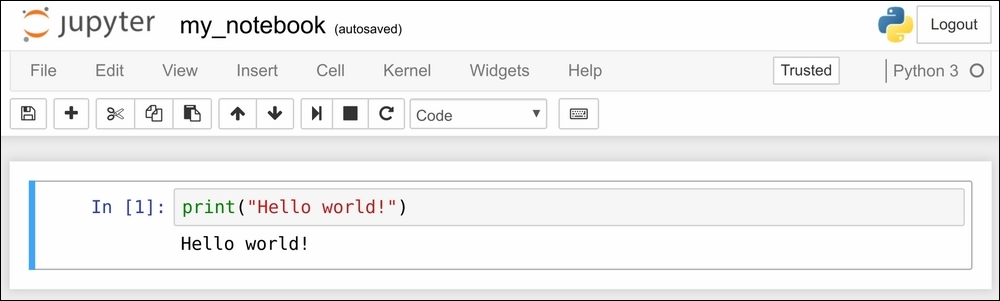A notebook contains a linear succession of cells and output areas. A cell contains Python code, in one or multiple lines. The output of the code is shown in the corresponding output area.

### Note

In this book, the prompt `>>>` means that you need to type everything that starts after it. The `>>>` characters themselves should not be typed.

2. Now, we do a simple arithmetic operation:

```>>> 2 + 2
4```

The result of the operation is shown in the output area. More precisely, the output area not only displays text that is printed by any command in the cell, but it also displays a text representation of the last returned object. Here, the last returned object is the result of `2 + 2`, that is, `4`.

3. In the next cell, we can recover the value of the last returned object with the `_` (underscore) special variable. In practice, it might be more convenient to assign objects to named variables such as in `myresult = 2 + 2`.

```>>> _ * 3
12```
4. IPython not only accepts Python code, but also shell commands. These commands are provided by the operating system. We first type `!` in a cell before typing the shell command. Here, assuming a Linux or macOS system, we get the list of all the notebooks in the current directory:

```>>> !ls
my_notebook.ipynb```

On Windows, one may replace `ls` by `dir`.

5. IPython comes with a library of magic commands. These commands are convenient shortcuts to common actions. They all start with `%` (the percent character). We can get the list of all magic commands with `%lsmagic`:

```>>> %lsmagic
Available line magics:
%alias  %alias_magic  %autocall  %automagic  %autosave  %bookmark  %cat  %cd  %clear  %colors  %config  %connect_info  %cp  %debug  %dhist  %dirs  %doctest_mode  %ed  %edit  %env  %gui  %hist  %history  %killbgscripts  %ldir  %less  %lf  %lk  %ll  %load  %load_ext  %loadpy  %logoff  %logon  %logstart  %logstate  %logstop  %ls  %lsmagic  %lx  %macro  %magic  %man  %matplotlib  %mkdir  %more  %mv  %notebook  %page  %pastebin  %pdb  %pdef  %pdoc  %pfile  %pinfo  %pinfo2  %popd  %pprint  %precision  %profile  %prun  %psearch  %psource  %pushd  %pwd  %pycat  %pylab  %qtconsole  %quickref  %recall  %rehashx  %reload_ext  %rep  %rerun  %reset  %reset_selective  %rm  %rmdir  %run  %save  %sc  %set_env  %store  %sx  %system  %tb  %time  %timeit  %unalias  %unload_ext  %who  %who_ls  %whos  %xdel  %xmode
Available cell magics:
%%!  %%HTML  %%SVG  %%bash  %%capture  %%debug  %%file  %%html  %%javascript  %%js  %%latex  %%markdown  %%perl  %%prun  %%pypy  %%python  %%python2  %%python3  %%ruby  %%script  %%sh  %%svg  %%sx  %%system  %%time  %%timeit  %%writefile

Automagic is ON, % prefix IS NOT needed for line magics.```

Cell magics have a `%%` prefix; they target entire code cells.

6. For example, the `%%writefile` cell magic lets us create a text file. This magic command accepts a filename as an argument. All the remaining lines in the cell are directly written to this text file. Here, we create a `test.txt` file and write `Hello world!` into it:

```>>> %%writefile test.txt
Hello world!
Writing test.txt
>>> # Let's check what this file contains.
with open('test.txt', 'r') as f:
Hello world!```
7. As we can see in the output of `%lsmagic`, there are many magic commands in IPython. We can find more information about any command by adding `?` after it. For example, to get some help about the `%run` magic command, we type `%run?` as shown here:

`>>> %run?`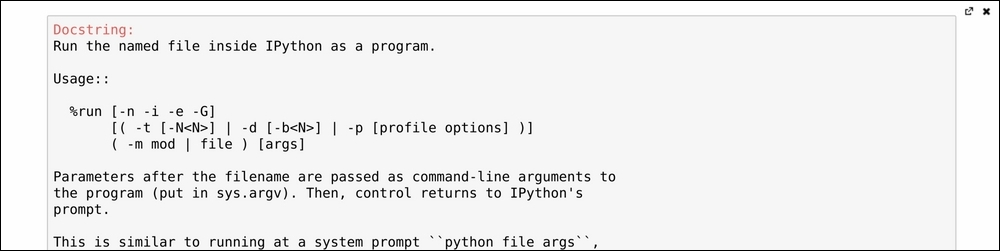The pager (a text area at the bottom of the screen) opens and shows the help of the `%run` magic command.

8. We covered the basics of IPython and the Notebook. Let's now turn to the rich display and interactive features of the Notebook. Until now, we have only created code cells (containing code). Jupyter supports other types of cells. In the Notebook toolbar, there is a drop-down menu to select the cell's type. The most common cell type after the code cell is the Markdown cell.

Markdown cells contain rich text formatted with Markdown, a popular plain text- formatting syntax. This format supports normal text, headers, bold, italics, hypertext links, images, mathematical equations in LaTeX (a typesetting system adapted to mathematics), code, HTML elements, and other features, as shown here: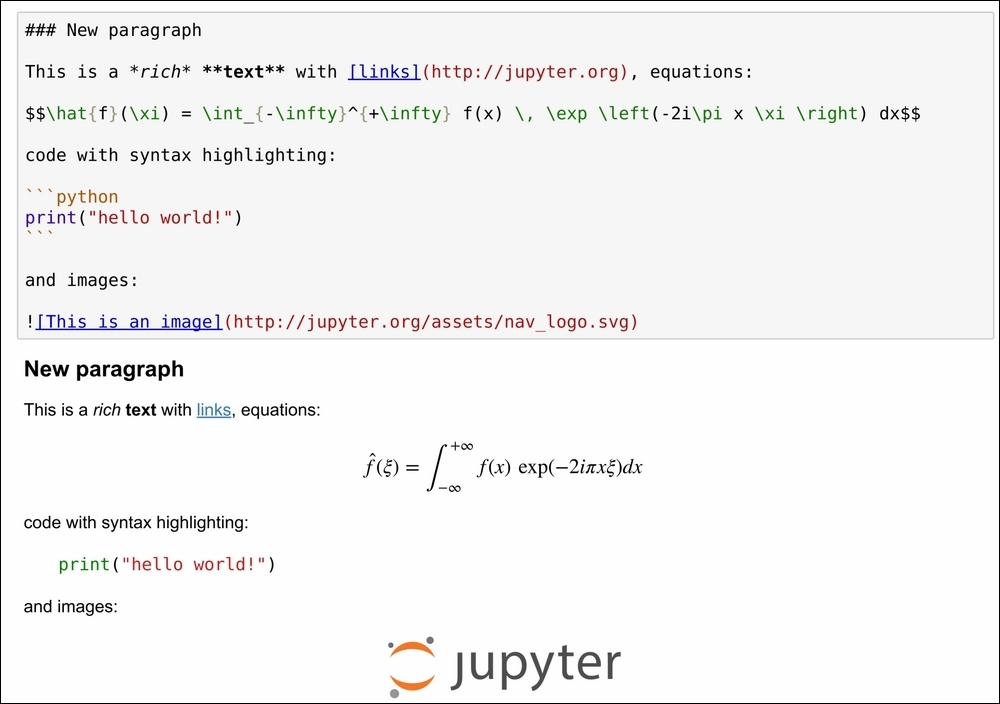Markdown cell

Running a Markdown cell (by pressing Shift + Enter, for example) displays the output, as shown in the bottom panel of the preceding screenshot.

By combining code cells and Markdown cells, we create a standalone interactive document that combines computations (code), text, and graphics.

9. The Jupyter Notebook also comes with a sophisticated display system that lets us insert rich web elements in the Notebook. Here, we show how to add HTML, Scalable Vector Graphics (SVG), and even YouTube videos in a notebook. First, we need to import some classes:

`>>> from IPython.display import HTML, SVG, YouTubeVideo`
10. We create an HTML table dynamically with Python, and we display it in the (HTML-based) notebook.

```>>> HTML('''
<table style="border: 2px solid black;">
''' +
''.join(['<tr>' +
''.join([f'<td>{row},{col}</td>'
for col in range(5)]) +
'</tr>' for row in range(5)]) +
'''
</table>
''')```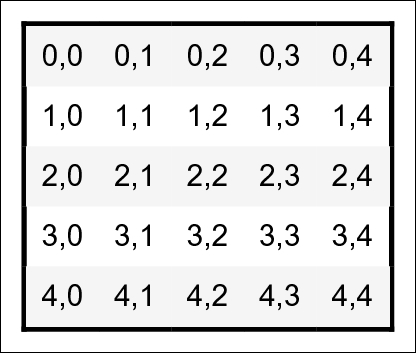11. Similarly, we create an SVG image dynamically:

```>>> SVG('''<svg width="600" height="80">''' +
''.join([f'''<circle
cx="{(30 + 3*i) * (10 - i)}"
cy="30"
r="{3. * float(i)}"
fill="red"
stroke-width="2"
stroke="black">
</circle>''' for i in range(10)]) +
'''</svg>''')```12. We display a YouTube video by giving its identifier to `YoutubeVideo`:

`>>> YouTubeVideo('VQBZ2MqWBZI')`### There's more...

Notebooks are saved as structured text files (JSON format), which makes them easily shareable. Here are the contents of a simple notebook:

```{
"cells": [
{
"cell_type": "code",
"execution_count": 1,
"outputs": [
{
"name": "stdout",
"output_type": "stream",
"text": [
"Hello world!\n"
]
}
],
"source": [
"print(\"Hello world!\")"
]
}
],
"nbformat": 4,
"nbformat_minor": 2
}```

Jupyter comes with a special tool, nbconvert, which converts notebooks to other formats such as HTML and PDF (https://nbconvert.readthedocs.io/en/stable/).

Another online tool, nbviewer (http://nbviewer.jupyter.org), allows us to render a publicly-available notebook directly in the browser.

We will cover many of these possibilities in subsequent chapters, notably in Chapter 3, Mastering the Jupyter Notebook.

There are other implementations of Jupyter Notebook frontends that offer different ways of interacting with the same notebook documents. JupyterLab, an IDE for interactive computing and data science, is the future of the Jupyter Notebook. It is introduced in Chapter 3, Mastering the Jupyter Notebook. nteract is a desktop application that lets the user open a notebook file by double-clicking on it, without using the Terminal and using a web browser. Hydrogen is a plugin of the Atom text editor that provides rich interactive capabilities when opening notebook files. Juno is a Jupyter Notebook client for iPad.

Here are a few references about the Notebook:

• The Getting started with exploratory data analysis in the Jupyt er Notebook recipe

• The Introducing JupyterLab recipe in Chapter 3, Mastering the Jupyter Notebook

## Getting started with exploratory data analysis in the Jupyter Notebook

In this recipe, we will give an introduction to IPython and Jupyter for data analysis. Most of the subject has been covered in the prequel of this book, Learning IPython for Interactive Computing and Data Visualization, Second Edition, Packt Publishing, but we will review the basics here.

We will download and analyze a dataset about attendance on Montreal's bicycle tracks. This example is largely inspired by a presentation from Julia Evans (available at https://github.com/jvns/talks/blob/master/2013-04-mtlpy/pistes-cyclables.ipynb). Specifically, we will introduce the following:

• Data manipulation with pandas

• Data visualization with Matplotlib

• Interactive widgets

### How to do it...

1. The very first step is to import the scientific packages we will be using in this recipe, namely NumPy, pandas, and Matplotlib. We also instruct Matplotlib to render the figures as inline images in the Notebook:

```>>> import numpy as np
import pandas as pd
import matplotlib.pyplot as plt
%matplotlib inline```

### Note

We can enable high-resolution Matplotlib figures on Retina display systems with the following commands:

```from IPython.display import set_matplotlib_formats
set_matplotlib_formats('retina')
```
2. Now, we create a new Python variable called `url` that contains the address to a Comma-separated Values (CSV) data file. This standard text-based file format is used to store tabular data:

```>>> url = ("https://raw.githubusercontent.com/"
"ipython-books/cookbook-2nd-data/"
"master/bikes.csv")```
3. pandas defines a `read_csv()` function that can read any CSV file. Here, we pass the URL to the file. pandas will automatically download the file, parse it, and return a DataFrame object. We need to specify a few options to make sure that the dates are parsed correctly:

```>>> df = pd.read_csv(url, index_col='Date',
parse_dates=True, dayfirst=True)```
4. The `df` variable contains a DataFrame object, a specific pandas data structure that contains 2D tabular data. The `head(n)` method displays the first `n` rows of this table. In the Notebook, pandas displays a DataFrame object in an HTML table, as shown in the following screenshot:

`>>> df.head(2)`Here, every row contains the number of bicycles on every track of the city, for every day of the year.

5. We can get some summary statistics of the table with the `describe()` method:

`>>> df.describe()`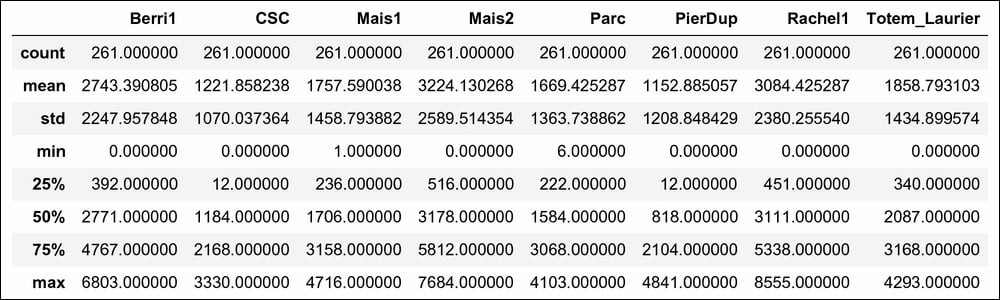6. Let's display some figures. We will plot the daily attendance of two tracks. First, we select the two columns, `Berri1` and `PierDup`. Then, we call the `plot()` method:

```>>> df[['Berri1', 'PierDup']].plot(figsize=(10, 6),
style=['-', '--'],
lw=2)```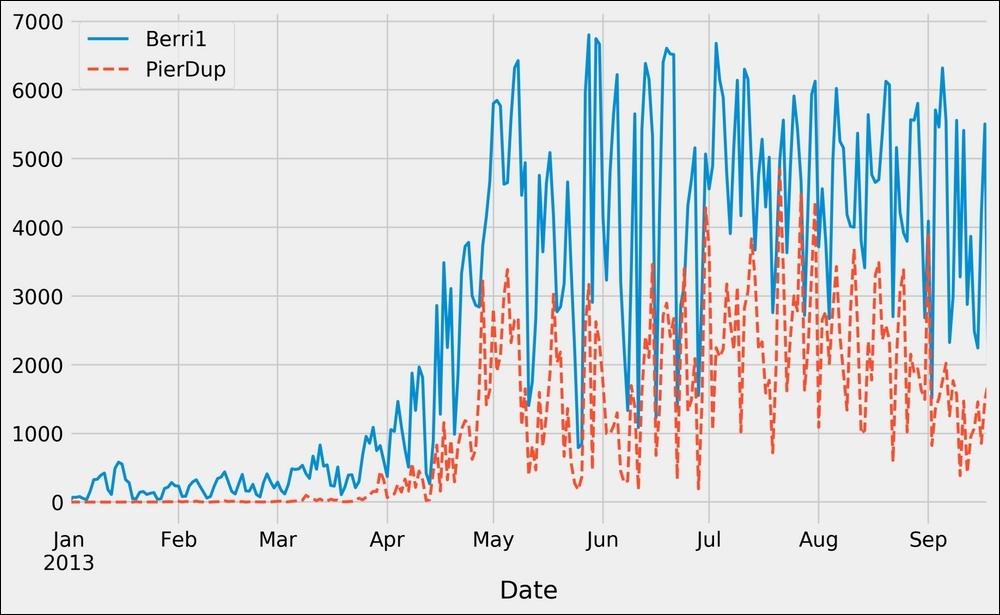7. Now, we move to a slightly more advanced analysis. We will look at the attendance of all tracks as a function of the weekday. We can get the weekday easily with pandas: the `index` attribute of the DataFrame object contains the dates of all rows in the table. This index has a few date-related attributes, including `weekday_name`:

```>>> df.index.weekday_name
Index(['Tuesday', 'Wednesday', 'Thursday', 'Friday',
'Saturday', 'Sunday', 'Monday', 'Tuesday',
...
'Friday', 'Saturday', 'Sunday', 'Monday',
'Tuesday', 'Wednesday'],
dtype='object', name='Date', length=261)```
8. To get the attendance as a function of the weekday, we need to group the table elements by the weekday. The `groupby()` method lets us do just that. We use `weekday` instead of `weekday_name` to keep the weekday order (Monday is `0`, Tuesday is `1`, and so on). Once grouped, we can sum all rows in every group:

```>>> df_week = df.groupby(df.index.weekday).sum()
>>> df_week```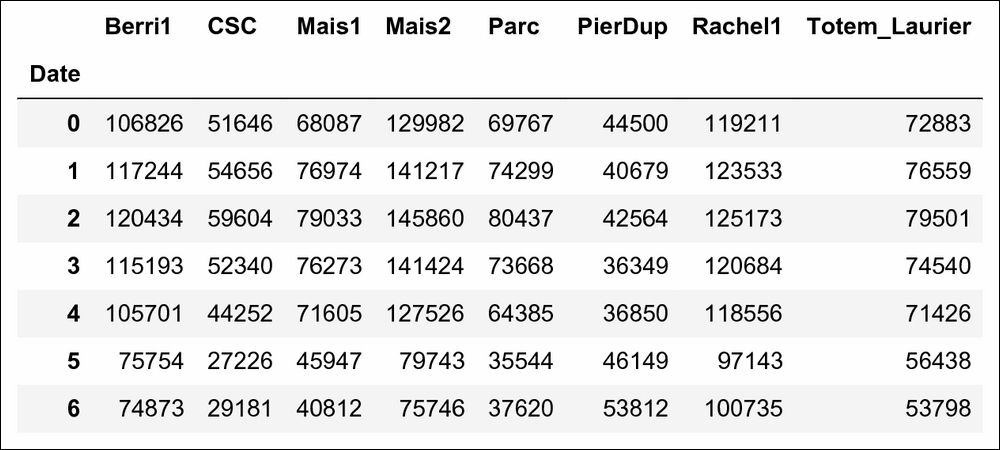9. We can now display this information in a figure. We create a Matplotlib figure, and we use the `plot()` method of DataFrame to create our plot:

```>>> fig, ax = plt.subplots(1, 1, figsize=(10, 8))
df_week.plot(style='-o', lw=3, ax=ax)
ax.set_xlabel('Weekday')
# We replace the labels 0, 1, 2... by the weekday
# names.
ax.set_xticklabels(
('Monday,Tuesday,Wednesday,Thursday,'
'Friday,Saturday,Sunday').split(','))
ax.set_ylim(0)  # Set the bottom axis to 0.```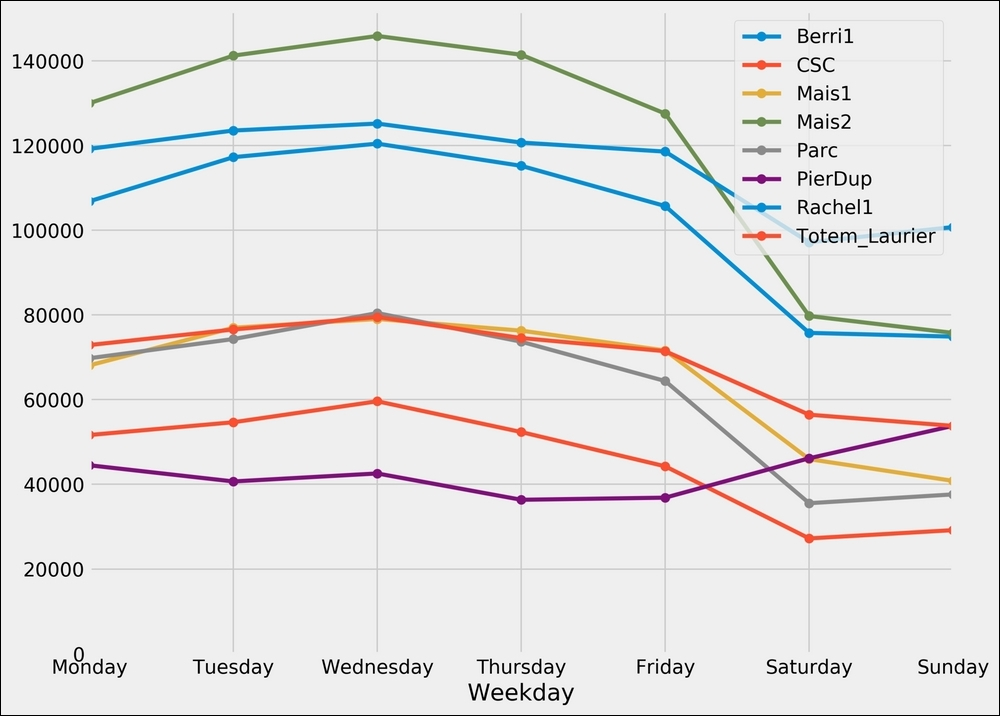10. Finally, let's illustrate the interactive capabilities of the Notebook. We will plot a smoothed version of the track attendance as a function of time (rolling mean). The idea is to compute the mean value in the neighborhood of any day. The larger the neighborhood, the smoother the curve. We will create an interactive slider in the Notebook to vary this parameter in real time in the plot. All we have to do is add the `@interact` decorator above our plotting function:

```>>> from ipywidgets import interact

@interact
def plot(n=(1, 30)):
fig, ax = plt.subplots(1, 1, figsize=(10, 8))
df['Berri1'].rolling(window=n).mean().plot(ax=ax)
ax.set_ylim(0, 7000)
plt.show()```### How it works...

To create Matplotlib figures, it is good practice to create a Figure (`fig`) and one or several Axes (subplots, `ax` object) objects with the `plt.subplots()` command. The `figsize` keyword argument lets us specify the size of the figure, in inches. Then, we call plotting methods directly on the Axes instances. Here, for example, we set the y limits of the axis with the `set_ylim()` method. If there are existing plotting commands, like the `plot()` method provided by pandas on DataFrame instances, we can pass the relevant Axis instance with the `ax` keyword argument.

### There's more...

pandas is the main data wrangling library in Python. Other tools and methods are generally required for more advanced analyses (signal processing, statistics, and mathematical modeling). We will cover these steps in the second part of this book, starting with Chapter 7, Statistical Data Analysis.

Here are some more references about data manipulation with pandas:

• The Introducing the multidimension al array in NumPy for fast array computations recipe

## Introducing the multidimensional array in NumPy for fast array computations

NumPy is the main foundation of the scientific Python ecosystem. This library offers a specific data structure for high-performance numerical computing: the multidimensional array. The rationale behind NumPy is the following: Python being a high-level dynamic language, it is easier to use but slower than a low-level language such as C. NumPy implements the multidimensional array structure in C and provides a convenient Python interface, thus bringing together high performance and ease of use. NumPy is used by many Python libraries. For example, pandas is built on top of NumPy.

In this recipe, we will illustrate the basic concepts of the multidimensional array. A more comprehensive coverage of the topic can be found in the book, Learning IPython for Interactive Computing and Data Visualization, Second Edition, Packt Publishing.

### How to do it...

1. Let's import the built-in `random` Python module and NumPy:

```>>> import random
import numpy as np```
2. We generate two Python lists, `x` and `y`, each one containing 1 million random numbers between 0 and 1:

```>>> n = 1000000
x = [random.random() for _ in range(n)]
y = [random.random() for _ in range(n)]
>>> x[:3], y[:3]
([0.926, 0.722, 0.962], [0.291, 0.339, 0.819])```
3. Let's compute the element-wise sum of all of these numbers: the first element of `x` plus the first element of `y`, and so on. We use a `for` loop in a list comprehension:

```>>> z = [x[i] + y[i] for i in range(n)]
z[:3]
[1.217, 1.061, 1.781]```
4. How long does this computation take? IPython defines a handy `%timeit` magic command to quickly evaluate the time taken by a single statement:

```>>> %timeit [x[i] + y[i] for i in range(n)]
101 ms ± 5.12 ms per loop (mean ± std. dev. of 7 runs,
10 loops each)```
5. Now we will perform the same operation with NumPy. NumPy works on multidimensional arrays, so we need to convert our lists to arrays. The `np.array()` function does just that:

```>>> xa = np.array(x)
ya = np.array(y)
>>> xa[:3]
array([ 0.926,  0.722,  0.962])```

The `xa` and `ya` arrays contain the exact same numbers that our original lists, `x` and `y`, contained. Those lists were instances of the list built-in class, while our arrays are instances of the `ndarray` NumPy class. These types are implemented very differently in Python and NumPy. In this example, we will see that using arrays instead of lists leads to drastic performance improvements.

6. To compute the element-wise sum of these arrays, we don't need to do a `for` loop anymore. In NumPy, adding two arrays means adding the elements of the arrays component-by-component. This is the standard mathematical notation in linear algebra (operations on vectors and matrices):

```>>> za = xa + ya
za[:3]
array([ 1.217,  1.061,  1.781])```

We see that the `z` list and the `za` array contain the same elements (the sum of the numbers in `x` and `y`).

### Note

Be careful not to use the `+` operator between vectors when they are represented as Python lists! This operator is valid between lists, so it would not raise an error and it could lead to subtle and silent bugs. In fact, `list1 + list2` is the concatenation of two lists, not the element-wise addition.

7. Let's compare the performance of this NumPy operation with the native Python loop:

```>>> %timeit xa + ya
1.09 ms ± 37.3 µs per loop (mean ± std. dev. of 7 runs,
1000 loops each)```

With NumPy, we went from 100 ms down to 1 ms to compute one million additions!

8. Now we will compute something else: the sum of all elements in `x` or `xa`. Although this is not an element-wise operation, NumPy is still highly efficient here. The pure Python version uses the built-in `sum()` function on an iterable. The NumPy version uses the `np.sum()` function on a NumPy array:

```>>> %timeit sum(x)  # pure Python
3.94 ms ± 4.44 µs per loop (mean ± std. dev. of 7 runs
100 loops each)
>>> %timeit np.sum(xa)  # NumPy
298 µs ± 4.62 µs per loop (mean ± std. dev. of 7 runs,
1000 loops each)```

We also observe a significant speedup here.

9. Let's perform one last operation: computing the arithmetic distance between any pair of numbers in our two lists (we only consider the first `1000` elements to keep computing times reasonable). First, we implement this in pure Python with two nested `for` loops:

```>>> d = [abs(x[i] - y[j])
for i in range(1000)
for j in range(1000)]
>>> d[:3]
[0.635, 0.587, 0.106]```
10. Now, we use a NumPy implementation, bringing out two slightly more advanced notions. First, we consider a two-dimensional array (or matrix). This is how we deal with the two indices, `i` and `j`. Second, we use broadcasting to perform an operation between a 2D array and 1D array. We will give more details in the How it works... section.

```>>> da = np.abs(xa[:1000, np.newaxis] - ya[:1000])
>>> da
array([[ 0.635,  0.587,  ...,  0.849,  0.046],
[ 0.431,  0.383,  ...,  0.646,  0.158],
...,
[ 0.024,  0.024,  ...,  0.238,  0.566],
[ 0.081,  0.033,  ...,  0.295,  0.509]])
>>> %timeit [abs(x[i] - y[j]) \
for i in range(1000) \
for j in range(1000)]
134 ms ± 1.79 ms per loop (mean ± std. dev. of 7 runs,
1000 loops each)
>>> %timeit np.abs(xa[:1000, np.newaxis] - ya[:1000])
1.54 ms ± 48.9 µs per loop (mean ± std. dev. of 7 runs
1000 loops each)```

Here again, we observe a significant speedup.

### How it works...

A NumPy array is a homogeneous block of data organized in a multidimensional finite grid. All elements of the array share the same data type, also called dtype (integer, floating-point number, and so on). The shape of the array is an n-tuple that gives the size of each axis.

A 1D array is a vector; its shape is just the number of components.

A 2D array is a matrix; its shape is (number of rows, number of columns).

The following diagram illustrates the structure of a 3D (3, 4, 2) array that contains 24 elements: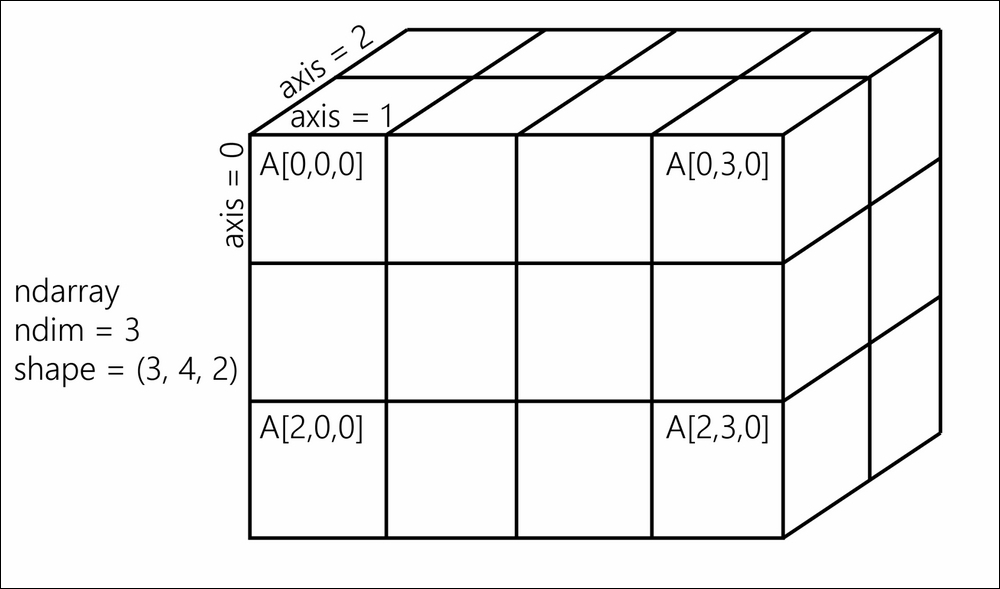A NumPy array

The slicing syntax in Python translates nicely to array indexing in NumPy. Also, we can add an extra dimension to an existing array, using `np.newaxis` in the index.

Element-wise arithmetic operations can be performed on NumPy arrays that have the same shape. However, broadcasting relaxes this condition by allowing operations on arrays with different shapes in certain conditions. Notably, when one array has fewer dimensions than the other, it can be virtually stretched to match the other array's dimension. This is how we computed the pairwise distance between any pair of elements in `xa` and `ya`.

How can array operations be so much faster than Python loops? There are several reasons, and we will review them in detail in Chapter 4, Profiling and Optimization. We can already say here that:

• In NumPy, array operations are implemented internally with C loops rather than Python loops. Python is typically slower than C because of its interpreted and dynamically-typed nature.

• The data in a NumPy array is stored in a contiguous block of memory in RAM. This property leads to more efficient use of CPU cycles and cache.

### There's more...

There's obviously much more to say about this subject. The prequel of this book, Learning IPython for Interactive Computing and Data Visualization, Second Edition, Packt Publishing, contains more details about basic array operations. We will use the array data structure routinely throughout this book. Notably, Chapter 4, Profiling and Optimization, covers advanced techniques of using NumPy arrays.

Here are some more references:

• The Getting started with exploratory data analysis in the Jupyter Notebook recipe

• The Understanding the internals of NumPy to avoid unne cessary array copying recipe in Chapter 4, Profiling and Optimization

## Creating an IPython extension with custom magic commands

Although IPython comes with a wide variety of magic commands, there are cases where we need to implement custom functionality in new magic commands. In this recipe, we will show how to create line and magic cells, and how to integrate them in an IPython extension.

### How to do it...

1. Let's import a few functions from the IPython magic system:

```>>> from IPython.core.magic import (register_line_magic,
register_cell_magic)```
2. Defining a new line magic is particularly simple. First, we create a function that accepts the contents of the line (except the initial `%`-prefixed name). The name of this function is the name of the magic. Then, we decorate this function with `@register_line_magic`:

```>>> @register_line_magic
def hello(line):
if line == 'french':
print("Salut tout le monde!")
else:
print("Hello world!")
>>> %hello
Hello world!
>>> %hello French
Salut tout le monde!```
3. Let's create a slightly more useful `%%csv` cell magic that parses a CSV string and returns a pandas DataFrame object. This time, the arguments of the function are the command's options and the contents of the cell:

```>>> import pandas as pd
from io import StringIO

@register_cell_magic
def csv(line, cell):
# We create a string buffer containing the
# contents of the cell.
sio = StringIO(cell)
# We use Pandas' read_csv function to parse
# the CSV string.
>>> %%csv
col1,col2,col3
0,1,2
3,4,5
7,8,9```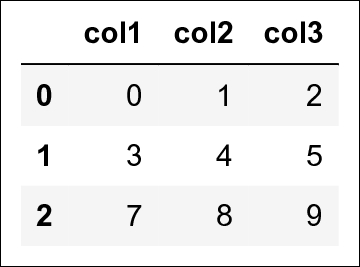We can access the returned object with `_`:

```>>> df = _
df.describe()```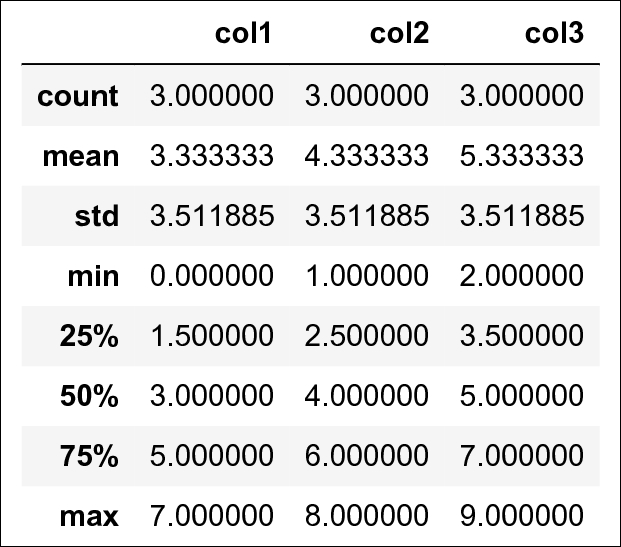4. The method we described is useful in an interactive session. If we want to use the same magic in multiple notebooks or if we want to distribute it, then we need to create an IPython extension that implements our custom magic command. The first step is to create a Python script (`csvmagic.py` here) that implements the magic. We also need to define a special function `load_ipython_extension(ipython)`:

```>>> %%writefile csvmagic.py
import pandas as pd
from io import StringIO

def csv(line, cell):
sio = StringIO(cell)

"""This function is called when the extension is
loaded. It accepts an IPython InteractiveShell
instance. We can register the magic with the
`register_magic_function` method of the shell
instance."""
ipython.register_magic_function(csv, 'cell')
Writing csvmagic.py```
5. Once the extension module is created, we need to import it into the IPython session. We can do this with the `%load_ext` magic command. Here, loading our extension immediately registers our `%%csv` magic function in the interactive shell:

```>>> %load_ext csvmagic
>>> %%csv
col1,col2,col3
0,1,2
3,4,5
7,8,9```### How it works...

An IPython extension is a Python module that implements the top-level `load_ipython_extension(ipython)` function. When the `%load_ext` magic command is called, the module is loaded and the `load_ipython_extension(ipython)` function is called. This function is passed the current `InteractiveShell` instance as an argument. This object implements several methods we can use to interact with the current IPython session.

#### The InteractiveShell class

An interactive IPython session is represented by a (singleton) instance of the `InteractiveShell` class. This object handles the history, interactive namespace, and most features available in the session.

Within an interactive shell, we can get the current `InteractiveShell` instance with the `get_ipython()` function.

The list of all methods of `InteractiveShell` can be found in the reference API (see the reference at the end of this recipe). The following are the most important attributes and methods:

• `user_ns`: The user namespace (a dictionary).

• `push()`: Push (or inject) Python variables in the interactive namespace.

• `ev()`: Evaluate a Python expression in the user namespace.

• `ex()`: Execute a Python statement in the user namespace.

• `run_cell()`: Run a cell (given as a string), possibly containing IPython magic commands.

• `safe_execfile()`: Safely execute a Python script.

• `system()`: Execute a system command.

• `write()`: Write a string to the default output.

• `write_err()`: Write a string to the default error output.

• `register_magic_function()`: Register a standalone function as an IPython magic function. We used this method in this recipe.

The Python extension module needs to be importable when using `%load_ext`. Here, our module is in the current directory. In other situations, it has to be in the Python path. It can also be stored in `~/.ipython/extensions`, which is automatically put in the Python path.

To ensure that our magic is automatically defined in our IPython profile, we can instruct IPython to load our extension automatically when a new interactive shell is launched. To do this, we have to open the `~/.ipython/profile_default/ipython_config.py` file and put `'csvmagic'` in the `c.InteractiveShellApp.extensions` list. The `csvmagic` module needs to be importable. It is common to create a Python package that implements an IPython extension, which itself defines custom magic commands.

### There's more...

Many third-party extensions and magic commands exist, for example the `%%cython` magic that allows one to write Cython code directly in a notebook.

Here are a few references:

• The Mastering IPython's configuration system recipe

## Mastering IPython's configuration system

The `traitlets` package (https://traitlets.readthedocs.io/en/stable/), originated from IPython, implements a powerful configuration system. This system is used throughout the project, but it can also be used by IPython extensions. It could even be used in entirely new applications.

In this recipe, we show how to use this system to write a configurable IPython extension. We will create a simple magic command that displays random numbers. This magic command comes with configurable parameters that can be set by the user in their IPython configuration file.

### How to do it...

1. We create an IPython extension in a `random_magics.py` file. Let's start by importing a few objects:

```>>> %%writefile random_magics.py

from traitlets import Int, Float, Unicode, Bool
from IPython.core.magic import (Magics, magics_class,
line_magic)
import numpy as np
Writing random_magics.py```
2. We create a `RandomMagics` class deriving from `Magics`. This class contains a few configurable parameters:

```>>> %%writefile random_magics.py -a

@magics_class
class RandomMagics(Magics):
text = Unicode(u'{n}', config=True)
max = Int(1000, config=True)
seed = Int(0, config=True)
Appending to random_magics.py```
3. We need to call the parent's constructor. Then, we initialize a random number generator with a seed:

```>>> %%writefile random_magics.py -a

def __init__(self, shell):
super(RandomMagics, self).__init__(shell)
self._rng = np.random.RandomState(
self.seed or None)
Appending to random_magics.py```
4. We create a `%random` line magic that displays a random number:

```>>> %%writefile random_magics.py -a

@line_magic
def random(self, line):
return self.text.format(
n=self._rng.randint(self.max))
Appending to random_magics.py```
5. Finally, we register that magic when the extension is loaded:

```>>> %%writefile random_magics.py -a

ipython.register_magics(RandomMagics)
Appending to random_magics.py```
6. Let's test our extension in the Notebook:

```>>> %load_ext random_magics
>>> %random
'430'
>>> %random
'305'```
7. Our magic command has a few configurable parameters. These variables are meant to be configured by the user in the IPython configuration file or in the console when starting IPython. To configure these variables in the Terminal, we can type the following command in a system shell:

```ipython --RandomMagics.text='Your number is {n}.' \
--RandomMagics.max=10 \
--RandomMagics.seed=1```

In that session, `%random` displays a string like `'Your number is 5.'`.

8. To configure the variables in the IPython configuration file, we open the `~/.ipython/profile_default/ipython_config.py` file and add the following line:

`c.RandomMagics.text = 'random {n}'`

After launching IPython, `%random` prints a string like random `652`.

### How it works...

IPython's configuration system defines several concepts:

• An user profile is a set of parameters, logs, and command history, which are specific to a user. A user can have different profiles when working on different projects. An `xxx` profile is stored in `~/.ipython/profile_xxx`, where `~` is the user's home directory.

• On Linux, the path should be `/home/yourname/.ipython/profile_xxx`

• On macOS, the path should be `/Users/yourname/.ipython/profile_xxx`

• On Windows, the path should be `C:\Users\YourName\.ipython\profile_xxx`

• A configuration object, or `Config`, is a special Python dictionary that contains key-value pairs. The `Config` class derives from Python's dict.

• The `HasTraits` class is a class that can have special trait attributes. Traits are sophisticated Python attributes that have a specific type and a default value. Additionally, when a trait's value changes, a callback function is automatically and transparently called. This mechanism allows a class to be notified whenever a trait attribute is changed.

• A `Configurable` class is the base class of all classes that want to benefit from the configuration system. A `Configurable` class can have configurable attributes. These attributes have default values specified directly in the class definition. The main feature of `Configurable` classes is that the default values of the traits can be overridden by configuration files on a class-by-class basis. Then, instances of the `Configurable` classes can change these values at leisure.

• A configuration file is a Python or JSON file that contains the parameters of the `Configurable` classes.

The `Configurable` classes and configuration files support an inheritance model. A `Configurable` class can derive from another `Configurable` class and override its parameters. Similarly, a configuration file can be included in another file.

#### Configurables

Here is a simple example of a `Configurable` class:

```from traitlets.config import Configurable
from traitlets import Float

class MyConfigurable(Configurable):
myvariable = Float(100.0, config=True)```

By default, an instance of the `MyConfigurable` class will have its `myvariable` attribute equal to `100.0`. Now, let's assume that our IPython configuration file contains the following lines:

```c = get_config()
c.MyConfigurable.myvariable = 123.```

Then, the `myvariable` attribute will default to `123`. Instances are free to change this default value after they are instantiated.

The `get_config()` function is a special function that is available in any configuration file.

Additionally, `Configurable` parameters can be specified in the command-line interface, as we saw in this recipe.

This configuration system is used by all IPython applications (notably console, Qt console, and notebook). These applications have many configurable attributes. You will find the list of these attributes in your profile's configuration files.

#### Magics

The `Magics` class derives from `Configurable` and can contain configurable attributes. Moreover, magic commands can be defined by methods decorated by `@line_magic` or `@cell_magic`. The advantage of defining class magics instead of function magics (as in the preceding recipe) is that we can keep a state between multiple magic calls (because we are using a class instead of a function).

### There's more...

Here are a few references:

• The Creating an IPython extension with custom magic commands recipe

## Creating a simple kernel for Jupyter

The architecture of Jupyter is language independent. The decoupling between the client and kernel makes it possible to write kernels in any language. The client communicates with the kernel via socket-based messaging protocols.

However, the messaging protocols are complex. Writing a new kernel from scratch is not straightforward. Fortunately, Jupyter brings a lightweight interface for kernel languages that can be wrapped in Python.

This interface can also be used to create an entirely customized experience in the Jupyter Notebook (or another client application, such as the console). Normally, Python code has to be written in every code cell; however, we can write a kernel for any domain-specific language. We just have to write a Python function that accepts a code string as input (the contents of the code cell), and sends text or rich data as output. We can also easily implement code completion and code inspection.

We can imagine many interesting interactive applications that go far beyond the original use cases of Jupyter. These applications might be particularly useful to nonprogrammer end users such as high school students.

In this recipe, we will create a simple graphing calculator. The calculator is transparently backed by NumPy and Matplotlib. We just have to write functions as `y = f(x)` in a code cell to get a graph of these functions.

### How to do it...

1. First, we create a `plotkernel.py` file. This file will contain the implementation of our custom kernel. Let's import a few modules:

```>>> %%writefile plotkernel.py

from ipykernel.kernelbase import Kernel
import numpy as np
import matplotlib.pyplot as plt
from io import BytesIO
import urllib, base64
Writing plotkernel.py```
2. We write a function that returns a base64-encoded PNG representation of a Matplotlib figure:

```>>> %%writefile plotkernel.py -a

def _to_png(fig):
"""Return a base64-encoded PNG from a
matplotlib figure."""
imgdata = BytesIO()
fig.savefig(imgdata, format='png')
imgdata.seek(0)
return urllib.parse.quote(
base64.b64encode(imgdata.getvalue()))
Appending to plotkernel.py```
3. Now, we write a function that parses a code string, which has the form `y=f(x)`, and returns a NumPy function. Here, `f` is an arbitrary Python expression that can use NumPy functions:

```>>> %%writefile plotkernel.py -a

_numpy_namespace = {n: getattr(np, n)
for n in dir(np)}
def _parse_function(code):
"""Return a NumPy function from a
string 'y=f(x)'."""
return lambda x: eval(code.split('=').strip(),
_numpy_namespace, {'x': x})
Appending to plotkernel.py```
4. For our new wrapper kernel, we create a class that derives from `Kernel`. There are a few metadata fields we need to provide:

```>>> %%writefile plotkernel.py -a

class PlotKernel(Kernel):
implementation = 'Plot'
implementation_version = '1.0'
language = 'python'  # will be used for
# syntax highlighting
language_version = '3.6'
language_info = {'name': 'plotter',
'mimetype': 'text/plain',
'extension': '.py'}
banner = "Simple plotting"
Appending to plotkernel.py```
5. In this class, we implement a `do_execute()` method that takes code as input and sends responses to the client:

```>>> %%writefile plotkernel.py -a

def do_execute(self, code, silent,
store_history=True,
user_expressions=None,
allow_stdin=False):

# We create the plot with matplotlib.
fig, ax = plt.subplots(1, 1, figsize=(6,4),
dpi=100)
x = np.linspace(-5., 5., 200)
functions = code.split('\n')
for fun in functions:
f = _parse_function(fun)
y = f(x)
ax.plot(x, y)
ax.set_xlim(-5, 5)

# We create a PNG out of this plot.
png = _to_png(fig)

if not silent:
# We send the standard output to the
# client.
self.send_response(
self.iopub_socket,
'stream', {
'name': 'stdout',
'data': ('Plotting {n} '
'function(s)'). \
format(n=len(functions))})

# We prepare the response with our rich
# data (the plot).
content = {
'source': 'kernel',

# This dictionary may contain
# different MIME representations of
# the output.
'data': {
'image/png': png
},

# We can specify the image size
# in the metadata field.
'image/png' : {
'width': 600,
'height': 400
}
}
}

# We send the display_data message with
# the contents.
self.send_response(self.iopub_socket,
'display_data', content)

# We return the exection results.
return {'status': 'ok',
'execution_count':
self.execution_count,
'user_expressions': {},
}
Appending to plotkernel.py```
6. Finally, we add the following lines at the end of the file:

```>>> %%writefile plotkernel.py -a

if __name__ == '__main__':
from ipykernel.kernelapp import IPKernelApp
IPKernelApp.launch_instance(
kernel_class=PlotKernel)
Appending to plotkernel.py```
7. Our kernel is ready! The next step is to indicate to Jupyter that this new kernel is available. To do this, we need to create a kernel spec `kernel.json` file in a subdirectory as follows:

```>>> %mkdir -p plotter/
>>> %%writefile plotter/kernel.json
{
"argv": ["python", "-m",
"plotkernel", "-f",
"{connection_file}"],
"display_name": "Plotter",
"name": "Plotter",
"language": "python"
}
Writing plotter/kernel.json```
8. We install the kernel:

```>>> !jupyter kernelspec install --user plotter
[InstallKernelSpec] Installed kernelspec plotter in
~/.local/share/jupyter/kernels/plotter```
9. The `plotter` kernel now appears in the list of kernels:

```>>> !jupyter kernelspec list
Available kernels:
bash         ~/.local/share/jupyter/kernels/bash
ir           ~/.local/share/jupyter/kernels/ir
plotter      ~/.local/share/jupyter/kernels/plotter
sagemath     ~/.local/share/jupyter/kernels/sagemath
...```

The `plotkernel.py` file needs to be importable by Python. For example, we could simply put it in the current directory.

10. Now, if we refresh the main Jupyter Notebook page (or after a restart of the Jupyter Notebook server if needed), we see that our Plotter kernel appears in the list of kernels: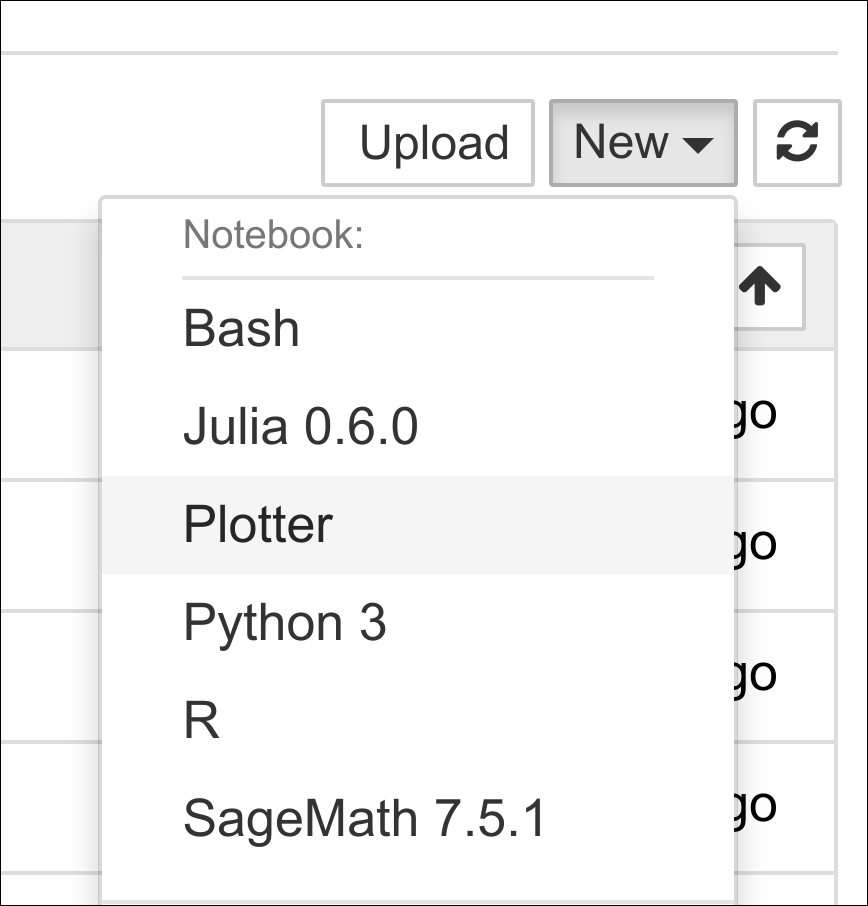Kernel list

11. Let's create a new notebook with the Plotter kernel. There, we can simply write mathematical equations under the form `y=f(x)`. The corresponding graph appears in the output area. Here is an example: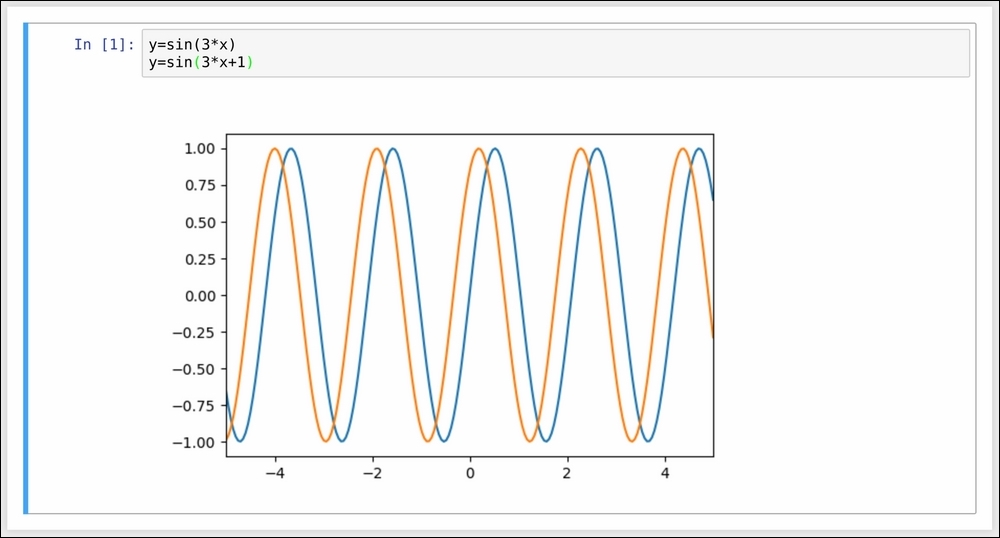Wrapper kernel

### How it works...

The kernel and client live in different processes. They communicate via messaging protocols implemented on top of network sockets. Currently, these messages are encoded in JSON, a structured, text-based document format.

Our kernel receives code from the client (the notebook, for example). The `do_execute()` function is called whenever the user sends a cell's code.

The kernel can send messages back to the client with the `self.send_response()` method:

• The first argument is the socket—here, the IOPub socket

• The second argument is the message type—here, `stream` to send back standard output or a standard error, or `display_data` to send back rich data

• The third argument is the contents of the message, represented as a Python dictionary

The data can contain multiple MIME representations: text, HTML, SVG, images, and others. It is up to the client to handle these data types. In particular, the Notebook client knows how to represent all these types in the browser.

The function returns execution results in a dictionary.

In this toy example, we always return an `ok` status. In production code, it would be a good idea to detect errors (syntax errors in the function definitions, for example) and return an error status instead.

All messaging protocol details can be found in the following section.

### There's more...

Wrapper kernels can implement optional methods, notably for code completion and code inspection. For example, to implement code completion, we need to write the following method:

```def do_complete(self, code, cursor_pos):
return {'status': 'ok',
'cursor_start': ...,
'cursor_end': ...,
'matches': [...]}```

This method is called whenever the user requests code completion when the cursor is at a given `cursor_pos` location in the code cell. In the method's response, the `cursor_start` and `cursor_end` fields represent the interval that code completion should overwrite in the output. The `matches` field contains the list of suggestions.

Here are a few references:

### About the Author

• ##### Cyrille Rossant

Cyrille Rossant, PhD, is a neuroscience researcher and software engineer at University College London. He is a graduate of École Normale Supérieure, Paris, where he studied mathematics and computer science. He has also worked at Princeton University and Collège de France. While working on data science and software engineering projects, he gained experience in numerical computing, parallel computing, and high-performance data visualization. He is the author of Learning IPython for Interactive Computing and Data Visualization, Second Edition, Packt Publishing.

Browse publications by this author

### Latest Reviews

(4 reviews total)